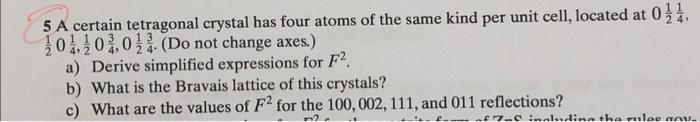# (Solved): with details please 5 A certain tetragonal crystal has four atoms of the same kind per unit cell, lo ...5 A certain tetragonal crystal has four atoms of the same kind per unit cell, located at $$0 \frac{1}{2} \frac{1}{4}$$, $$\frac{1}{2} 0 \frac{1}{4}, \frac{1}{2} 0 \frac{3}{4}, 0 \frac{1}{2} \frac{3}{4}$$. (Do not change axes.) a) Derive simplified expressions for $$F^{2}$$. b) What is the Bravais lattice of this crystals? c) What are the values of $$F^{2}$$ for the $$100,002,111$$, and 011 reflections?